# Physics 4 March 30 2017 P 3 Challenge

• Slides: 14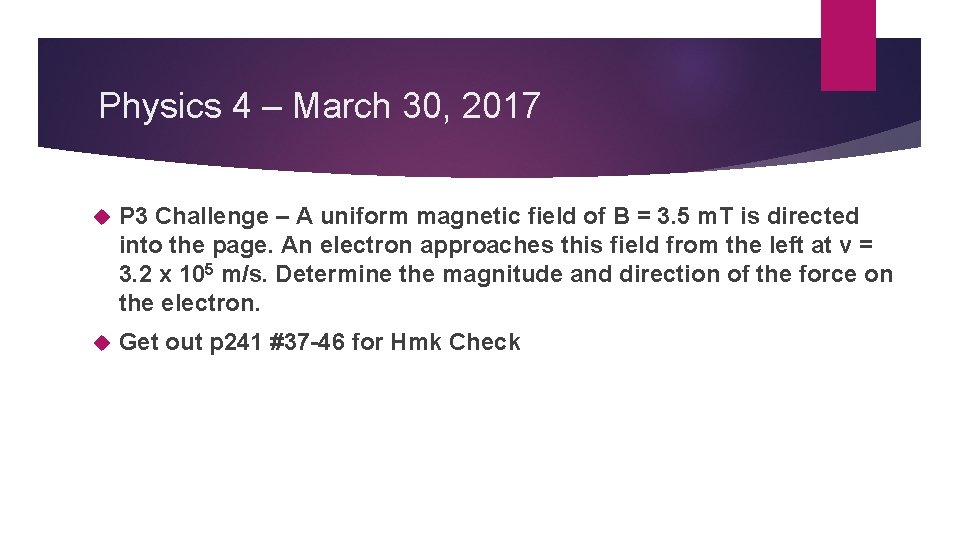Physics 4 – March 30, 2017 P 3 Challenge – A uniform magnetic field of B = 3. 5 m. T is directed into the page. An electron approaches this field from the left at v = 3. 2 x 105 m/s. Determine the magnitude and direction of the force on the electron. Get out p 241 #37 -46 for Hmk CheckObjectives/Agenda/Assignment Objective: Ch 5. 4 Magnetism (finish) Ch 11. 1 Induction Agenda: Assignment: p 442 #1 -13 Magnetic force on current carrying wire Force between two current carrying wires HMK review Induced motional emf Magnetic flux Faraday’s Law Lenz’s LawRest of Year Overview March 30 – 11. 1 Induction April 3 - 11. 2 Alternating Current April 6 – Review / Buffer April 7 Review April 11 – Electromagnetism Test April 13 – B. 2 Thermodynamics April 18 – B. 3 Fluids (no test for Opt B) April 20 – 7. 1 Radioactivity and 7. 2 Nuclear reactions April 25 – 7. 3 Particle Physics April 27 – 12. 1 Matter and Radiation April 28 – 12. 2 Nuclear Physics May 2 – Review May 4 – Nuclear Test May 8 – Review May 10 -11 – Senior Finals (IB practice) May 15 – IB Physics Papers 1 and 2 May 16 – IB Physics Paper 3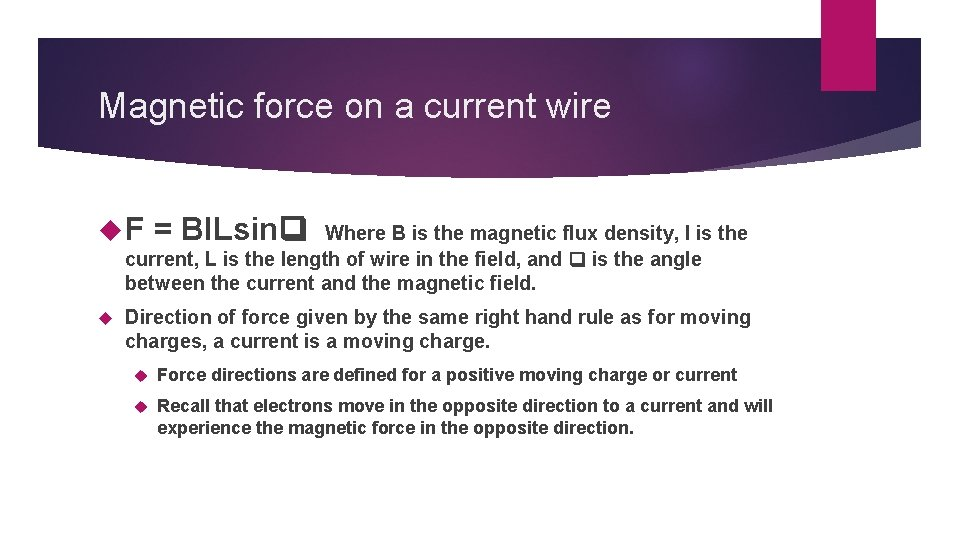Magnetic force on a current wire F = BILsin Where B is the magnetic flux density, I is the current, L is the length of wire in the field, and is the angle between the current and the magnetic field. Direction of force given by the same right hand rule as for moving charges, a current is a moving charge. Force directions are defined for a positive moving charge or current Recall that electrons move in the opposite direction to a current and will experience the magnetic force in the opposite direction.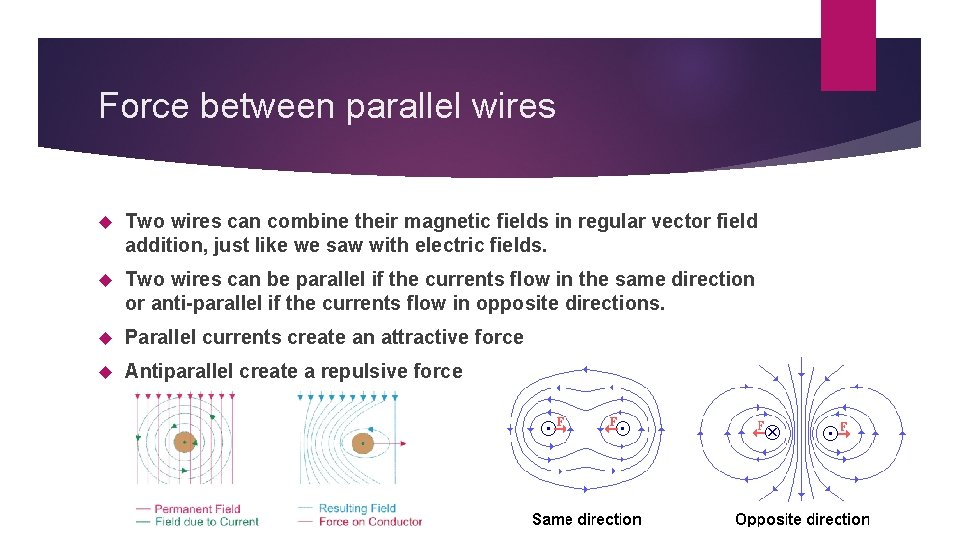Force between parallel wires Two wires can combine their magnetic fields in regular vector field addition, just like we saw with electric fields. Two wires can be parallel if the currents flow in the same direction or anti-parallel if the currents flow in opposite directions. Parallel currents create an attractive force Antiparallel create a repulsive forceDefinition of the Ampere Remember we use the ampere as the fundamental SI base units for all things electrical. Why? Because it can be standardized through the magnetic force between current carrying wires: If the force on a 1 m length of two wires that are 1 m apart and carrying equal currents is 2 x 10 -7 N, then the current in each wire is defined to be 1 Ampere.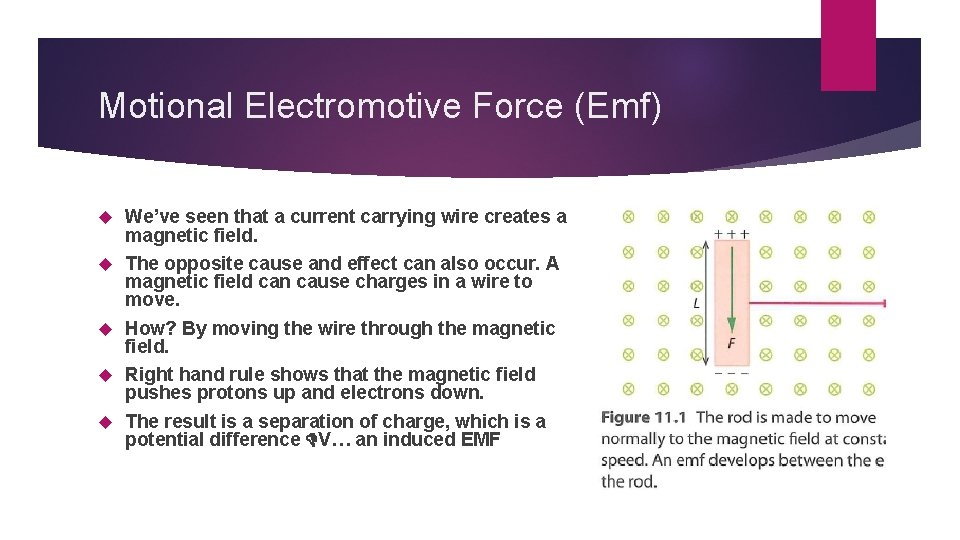Motional Electromotive Force (Emf) We’ve seen that a current carrying wire creates a magnetic field. The opposite cause and effect can also occur. A magnetic field can cause charges in a wire to move. How? By moving the wire through the magnetic field. Right hand rule shows that the magnetic field pushes protons up and electrons down. The result is a separation of charge, which is a potential difference V… an induced EMFMagnitude of induced EMF = Bv. L where B is the magnetic field (T), v is the speed of the wire moving through the field, and L is the length of the wire located within the field. Called a motional emf because it requires motion to create the emf. If there are multiple loops of wire, you can multiply this emf by the number of loops, N, to find the overall emf of the solenoid. = Bv. LN Both of these forms are in the data packetPower of Induced emf Connecting this emf to a simple circuit, you can determine the current through a resistor, R. IR = Bv. L then solve for I = Bv. L/R The now current carrying wire generates a force F = BIL = B(Bv. L/R)L = B 2 v. L 2 /R Recall Power = Fv = W/t Power of the force generated by the induced emf: P = (B 2 v. L 2 /R)v = B 2 v 2 L 2 /R Remembering before in Ch 5. 2. = Bv. L , P= 2/R This is equivalent to P = V 2/R we sawMagnetic Flux, Motion is required for an induced emf. We can move the magnetic field through a stationary solenoid as shown. Equivalent to moving a wire through a magnetic field. Only relative motion is required. Observation of this system show larger currents with: Greater relative speed Stronger magnetic field Higher number of turns Greater area of loop Max value when magnet and turns are perpendicular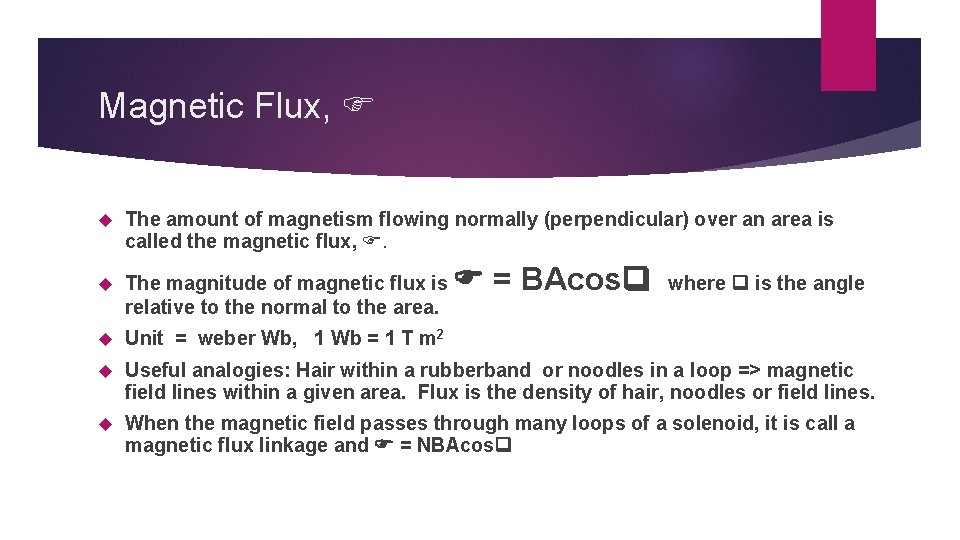Magnetic Flux, The amount of magnetism flowing normally (perpendicular) over an area is called the magnetic flux, . The magnitude of magnetic flux is relative to the normal to the area. Unit = weber Wb, 1 Wb = 1 T m 2 Useful analogies: Hair within a rubberband or noodles in a loop => magnetic field lines within a given area. Flux is the density of hair, noodles or field lines. When the magnetic field passes through many loops of a solenoid, it is call a magnetic flux linkage and = NBAcos = BAcos where is the angle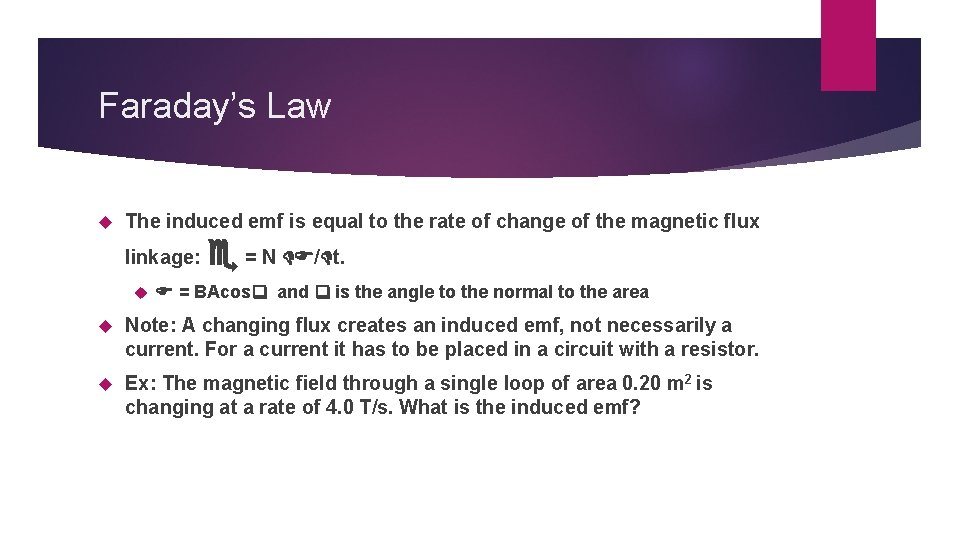Faraday’s Law The induced emf is equal to the rate of change of the magnetic flux linkage: = N / t. = BAcos and is the angle to the normal to the area Note: A changing flux creates an induced emf, not necessarily a current. For a current it has to be placed in a circuit with a resistor. Ex: The magnetic field through a single loop of area 0. 20 m 2 is changing at a rate of 4. 0 T/s. What is the induced emf?Lenz’s Law The induced emf will be in such a direction as to oppose the change in the magnetic flux that created the current. Consider an increase as directed in the direction of the magnetic field. Consider a decrease as directed opposite the magnetic field. Pretend the loop is a solenoid with one turn and use the Right Hand Grip rule to determine the direction of a magnetic field for the loop that will oppose the “change direction” Consider a loop in a field directed into board a) increasing, b) decreasing Consider a loop in a field directed out of board a) increasing b) decreasing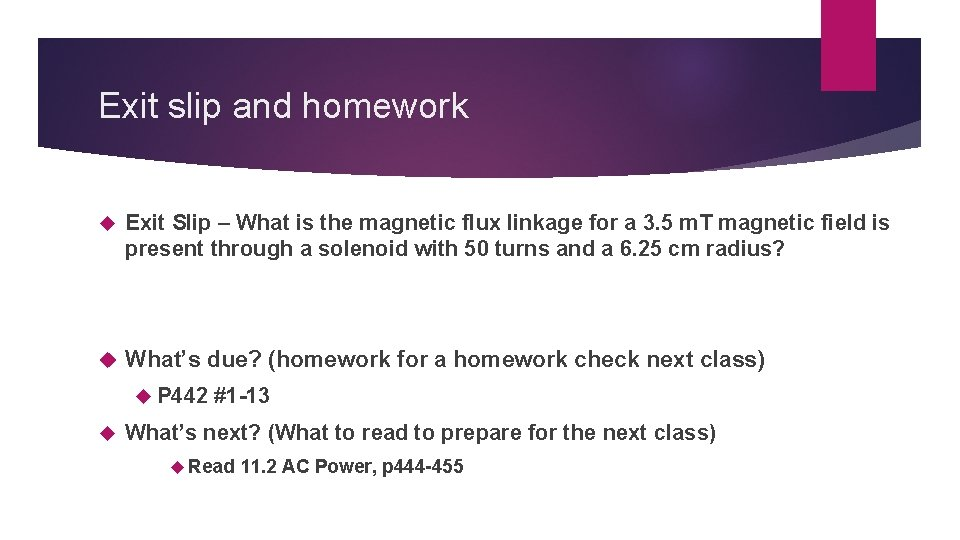Exit slip and homework Exit Slip – What is the magnetic flux linkage for a 3. 5 m. T magnetic field is present through a solenoid with 50 turns and a 6. 25 cm radius? What’s due? (homework for a homework check next class) P 442 #1 -13 What’s next? (What to read to prepare for the next class) Read 11. 2 AC Power, p 444 -455